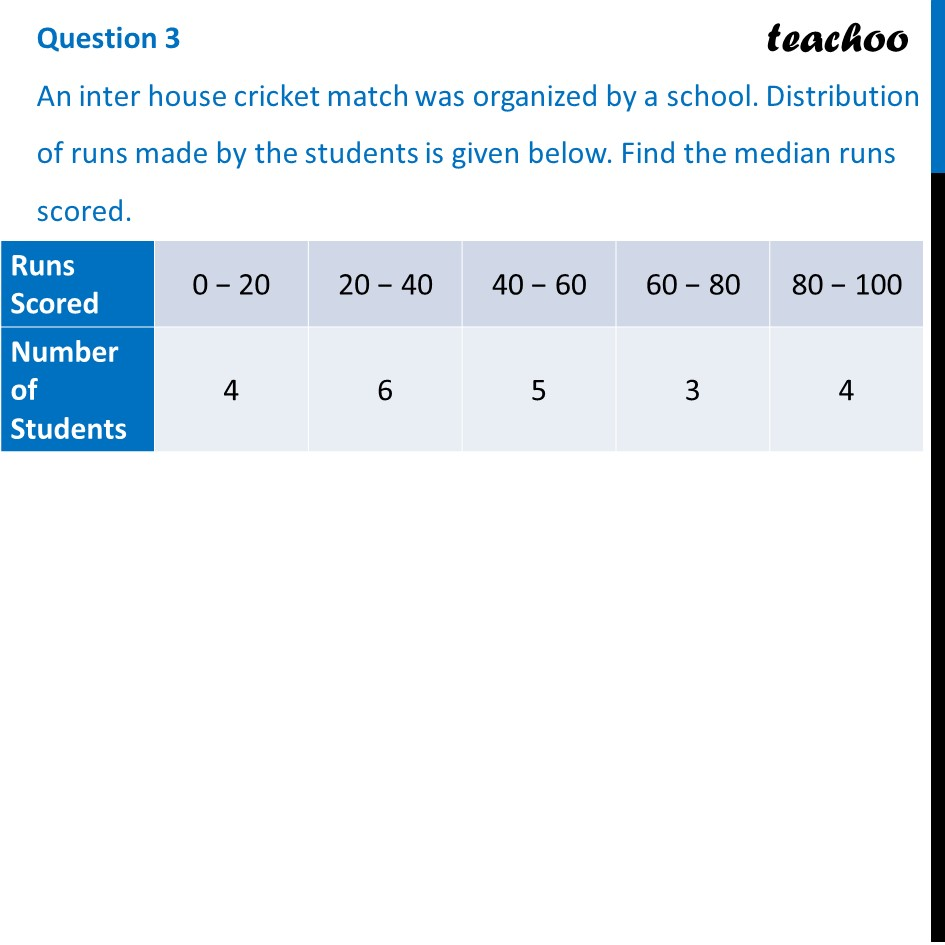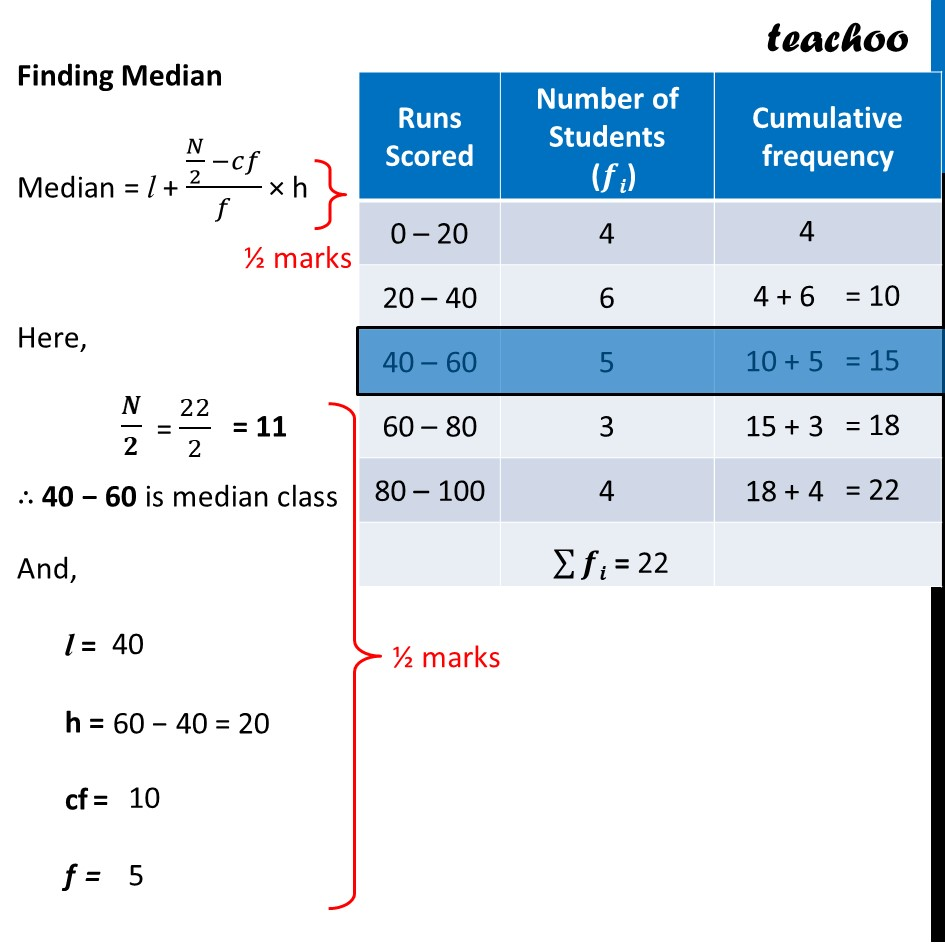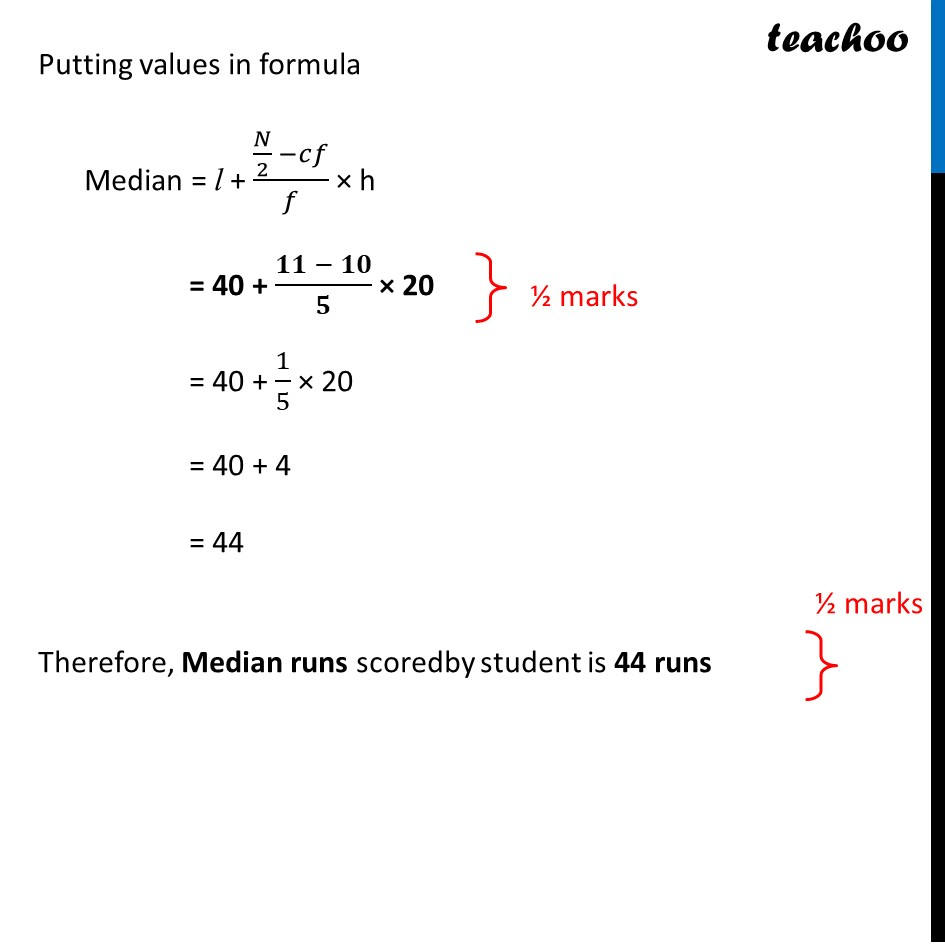CBSE Class 10 Sample Paper for 2022 Boards - Maths Basic [Term 2]

Class 10
Solutions of Sample Papers for Class 10 Boards

## An inter house cricket match was organized by a school. Distribution of runs made by the students is given below. Find the median runs scored.

This question is similar to Ex 14.3, 1 Chapter 4 Class 10 - StatisticsLearn in your speed, with individual attention - Teachoo Maths 1-on-1 Class

### Transcript

An inter house cricket match was organized by a school. Distribution of runs made by the students is given below. Find the median runs scored. Finding Median Median = l + (𝑁/2 −𝑐𝑓)/𝑓 × h Here, 𝑵/𝟐 ∴ 40 − 60 is median class And, l = h = cf = f = Putting values in formula Median = l + (𝑁/2 −𝑐𝑓)/𝑓 × h = 40 + (𝟏𝟏 − 𝟏𝟎)/𝟓 × 20 = 40 + 1/5 × 20 = 40 + 4 = 44 Therefore, Median runs scoredby student is 44 runs